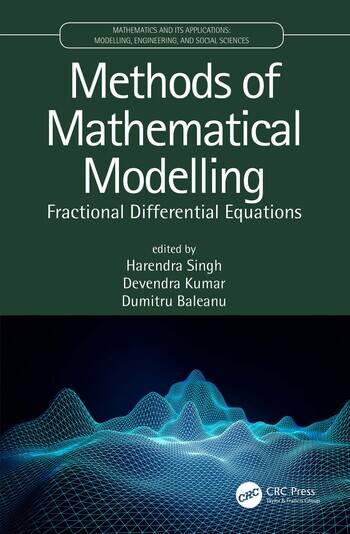Methods of Mathematical Modelling: Fractional Differential Equations

1st Edition

Harendra Singh, Devendra Kumar, Dumitru Baleanu

CRC Press
Published September 30, 2019
Reference - 238 Pages - 75 B/W Illustrations
ISBN 9780367220082 - CAT# 304062
Series: Mathematics and its Applications

For Instructors Request Inspection Copy

was \$169.95

USD\$135.96

SAVE ~\$33.99

FREE Standard Shipping!

Preview

Summary

This book features original research articles on the topic of mathematical modelling and fractional differential equations. The contributions, written by leading researchers in the field, consist of chapters on classical and modern dynamical systems modelled by fractional differential equations in physics, engineering, signal processing, fluid mechanics, and bioengineering, manufacturing, systems engineering, and project management.

The book offers theory and practical applications for the solutions of real-life problems and will be of interest to graduate level students, educators, researchers, and scientists interested in mathematical modelling and its diverse applications.

Features

• Presents several recent developments in the theory and applications of fractional calculus
• Includes chapters on different analytical and numerical methods dedicated to several mathematical equations
• Develops methods for the mathematical models which are governed by fractional differential equations
• Provides methods for models in physics, engineering, signal processing, fluid mechanics, and bioengineering
• Discusses real-world problems, theory, and applications

Instructors

We provide complimentary e-inspection copies of primary textbooks to instructors considering our books for course adoption.